Next: General Analysis Up: Time-Dependent Perturbation Theory Previous: Time-Dependent Perturbation Theory

# Introduction

Suppose that the Hamiltonian of a given quantum mechanical system can be written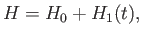(8.1)

where the unperturbed Hamiltonian,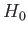, does not depend on time explicitly, and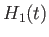is a small time-dependent perturbation. In the following, it is assumed that we are able to calculate the eigenkets and eigenvalues of the unperturbed Hamiltonian exactly. These are denoted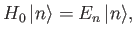(8.2)

where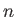is an integer. We know that if the system is initially in one of the eigenstates ofthen, in the absence of the external perturbation, it remains in that state for ever. (See Section 3.5.) However, the presence of a small time-dependent perturbation can, in principle, give rise to a finite probability that a system initially in some eigenstate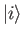of the unperturbed Hamiltonian is found in some other eigenstate at a subsequent time (becauseis no longer an exact eigenstate of the total Hamiltonian). In other words, a time-dependent perturbation allows the system to make transitions between its unperturbed energy eigenstates. Let us investigate such transitions.Next: General Analysis Up: Time-Dependent Perturbation Theory Previous: Time-Dependent Perturbation Theory
Richard Fitzpatrick 2016-01-22